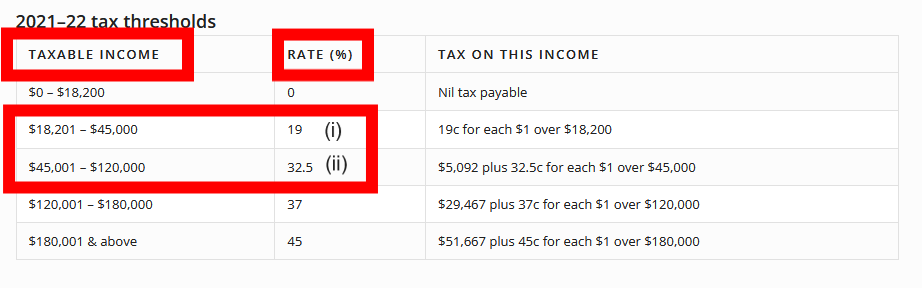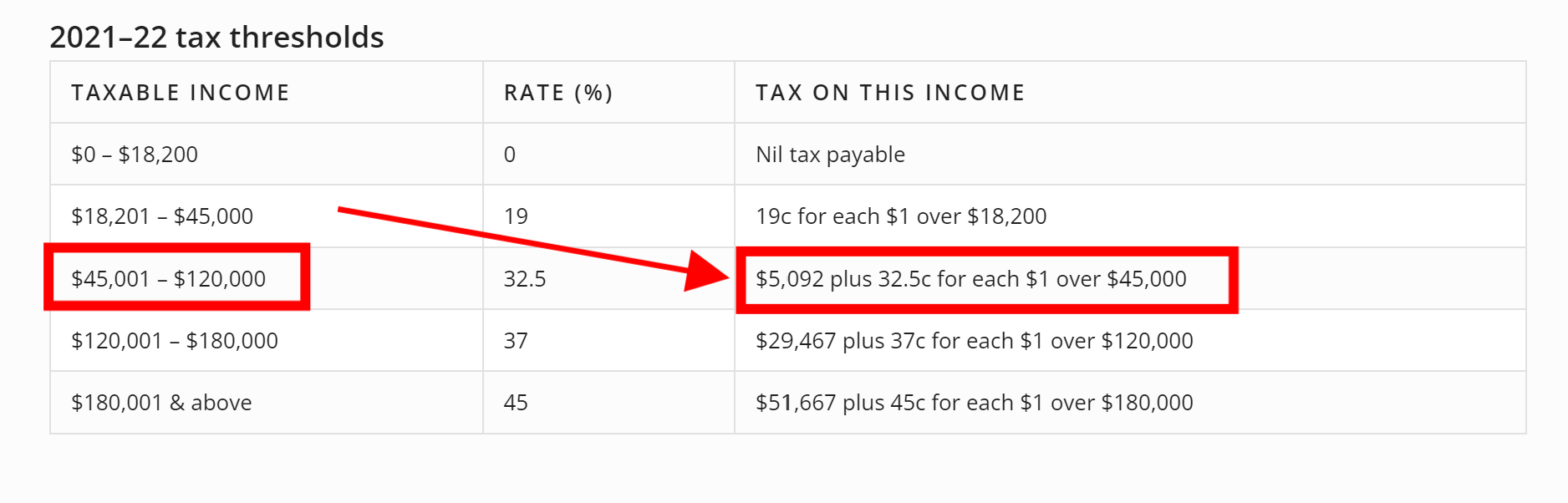## What is the tax rate on a capital gain?

It is a common misconception that there is a flat tax rate for a capital gain; however, there is no set tax rate on capital gain earnings. The tax is calculated on the individual’s marginal tax rates and will depend on what tax bracket the taxpayer currently falls into. Often a capital gain will overlap into multiple tax brackets, so may be taxed at two different rates.

I will use a basic example to demonstrate how the tax is calculated on a capital gain and income overlapping into multiple tax brackets. For further information on the marginal tax brackets, please refer to our Rates & Thresholds section.

### Example

Linda had a net capital gain on selling some shares of \$10,000. She is also employed and has a gross part-time wage of \$45,000 less \$5,000 of allowable tax deductions. Her income would be taxed as follows and will demonstrate this in order easier for understanding purposes:

Gross wage – \$45,000

Less allowable deductions – (\$5,000)

Total assessable income of \$40,000 (45,000 – 5,000) – excluding capital gain income

By referring to the tax tables (note these rates exclude Medicare Levy), you will see that any income generated up to \$45,000 is taxed at 19% (i) and then above this is taxed at 32.5% – up to \$120,000 (ii).Based on this, Linda can still earn an additional \$5,000 on top of her net wages, which will be taxed at 19%, but then any income after this will be taxed at 32.5%.

Current tax on her net wage is (\$40,000 (assessable wage income) LESS the tax-free threshold of \$18,200) x 19%.

= (40,000-18,200) x .19

= 21,800 x .19

= \$4,142 Tax on assessable income portion (excluding Medicare Levy)

From here, we can add the tax on the capital gain. \$5,000 of the income will be taxed at 19%, and the remaining \$5,000 will be at 32.5% (Total net capital gain of \$10,000).

= (5,000 x .19) + (5,000 x .325)

= 950 + 1,625

= 2,575 Tax on the capital gain portion (excluding Medicare Levy)

Total tax liability from Linda would therefor be the tax portion on her assessable income plus the tax portion on her capital gain.

= (4,142 + 2,575)

= \$6,717 Tax Payable (excluding Medicare Levy)

The actual way the tax calculation works is that your entire income is pooled together in the tax return, no matter what type of income you receive. From here, your allowable deductions are reduced to arrive at your ‘taxable income’ amount. Tax is then calculated on the total amount (rather than being broken down in our example for demonstration purposes only).

We can test this result above using the tax table calculation method.

In this instance, we can pool all our income together (gross wage plus the capital gain):

= 45,000 + 10,000

= 55,000 Assessable Income

Less allowable deductions:

= 55,000 – 5,000

= 50,000 Taxable Income

Using the tax tables, we need to calculate \$5,092 plus 32.5c for each \$1 over \$45,000 (see the image below):= 5,092 + (.325 x (50,000 – 45,000)) – Where 50,000 is our total Assessable Income

= 5,092 + (.325 x 5,000)

= 5,092 + 1,625

= \$6,717 Tax Payable (excluding Medicare Levy)

You will see that this also balances with or original example above.

To summarise, the amount of capital gains tax payable will all depend on the individuals’ earnings from other sources less their allowable deductions to determine what tax bracket they currently fall into. From here, the tax can be calculated either by apportioning in the correct tax bracket (as per example one) or using the tax table method (example two). Obviously, there are various other factors that would need to be taken into consideration (for example, if any CGT discounts or exemptions apply), however, hopefully, this gives you a greater understanding of how it applies.

## Why is my ATO refund less than last year?

Here are some of the instances that may cause your ATO refund to be lower than the previous year:

## 5 Tax tips to maximise your refund as a salary & wage employee

Here are a hand full of strategies that may help you maximise your tax refund in the upcoming financial year: